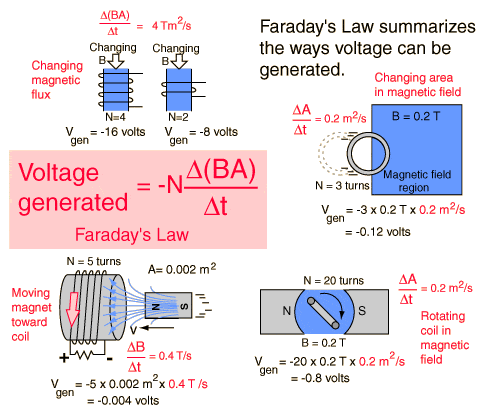# Designing a lundell(clawpole ) Type Generator

1. The problem statement, all variables and given/known data
I want to know the affect of stator design on bicycle dynamo performance using magnetic circuits, and power output. The bicycle dynamo is a single phase AC generator with permanent magnets on its rotor (mounted on hubshell) and a lundell type armature(mounted on bike axle). At 30rpm, the dynamo is expected give out 2.4W at 3V. Load is 15ohm.

2. Relevant equations
I've tried using Faraday' law, Voltage generated=-N(change in flux & area)over time, but I keep getting larger results than expected.

My attempt at applying:Total B of the magnet is: 4000mT, so for this voltage estimation I am assuming perfect coupling of field through the stators
The coil has a cross area of 483.05mm^2 and 320 turns.
There are 28 poles, so I assume the coil experiences a field change 14 times per second at 30rpm.

So Voltage generated = -(320)(4000mT)(483.05mm^2*14) = 8.65V

How do you factor in the 15 ohm load into the basic equation?

3. The attempt at a solution
Aside from the attempt in the previous section, I'm stuck. Would appreciate links or recommendations of reference if some reading is in order.

Thank you,
Derek

Last edited:

I suspect that you cannot assume perfect magnetic coupling. This type of simple alternator lighting generator generally relies on having a significant equivalent series inductance. At low speeds the inductive reactance is negligible, but at higher speeds the increasing frequency makes the reactance increase until it dominates the total circuit impedance.

The net effect is to stabilise the output voltage seen by the lamps against speed variation, so that for instance bulbs are less likely to blow when descending a hill at high speed. Unfortunately, this makes the loading (your 15 ohms) fairly critical: the correct wattage bulbs must always be fitted, and if two bulbs are used in parallel, failure of one tends to provoke rapid failure of the other.

I think you would need to estimate the generator leakage inductance (it's too long since I did magnetics to advise you about that!), work out its reactance at the working frequency, and go on to find the total impedance including the 15 ohm load. Apply your calculated generator voltage across the total impedance to find the true load current.

Last edited:

### The Physics Forums Way

We Value Quality
• Topics based on mainstream science
• Proper English grammar and spelling
We Value Civility
• Positive and compassionate attitudes
• Patience while debating
We Value Productivity
• Disciplined to remain on-topic
• Recognition of own weaknesses
• Solo and co-op problem solving